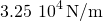15 Oscillations

15.6 Forced Oscillations

Learning Objectives

By the end of this section, you will be able to:

• Define forced oscillations
• List the equations of motion associated with forced oscillations
• Explain the concept of resonance and its impact on the amplitude of an oscillator
• List the characteristics of a system oscillating in resonance

Sit in front of a piano sometime and sing a loud brief note at it with the dampers off its strings ((Figure)). It will sing the same note back at you—the strings, having the same frequencies as your voice, are resonating in response to the forces from the sound waves that you sent to them. This is a good example of the fact that objects—in this case, piano strings—can be forced to oscillate, and oscillate most easily at their natural frequency. In this section, we briefly explore applying a periodic driving force acting on a simple harmonic oscillator. The driving force puts energy into the system at a certain frequency, not necessarily the same as the natural frequency of the system. Recall that the natural frequency is the frequency at which a system would oscillate if there were no driving and no damping force.

Most of us have played with toys involving an object supported on an elastic band, something like the paddle ball suspended from a finger in (Figure). Imagine the finger in the figure is your finger. At first, you hold your finger steady, and the ball bounces up and down with a small amount of damping. If you move your finger up and down slowly, the ball follows along without bouncing much on its own. As you increase the frequency at which you move your finger up and down, the ball responds by oscillating with increasing amplitude. When you drive the ball at its natural frequency, the ball’s oscillations increase in amplitude with each oscillation for as long as you drive it. The phenomenon of driving a system with a frequency equal to its natural frequency is called resonance. A system being driven at its natural frequency is said to resonate. As the driving frequency gets progressively higher than the resonant or natural frequency, the amplitude of the oscillations becomes smaller until the oscillations nearly disappear, and your finger simply moves up and down with little effect on the ball.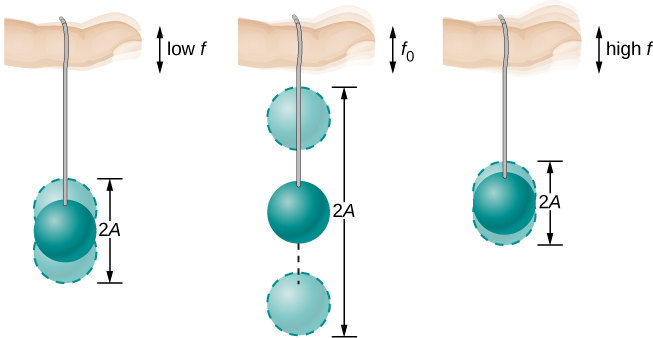Figure 15.29 The paddle ball on its rubber band moves in response to the finger supporting it. If the finger moves with the natural frequency     of the ball on the rubber band, then a resonance is achieved, and the amplitude of the ball’s oscillations increases dramatically. At higher and lower driving frequencies, energy is transferred to the ball less efficiently, and it responds with lower-amplitude oscillations.

Consider a simple experiment. Attach a mass m to a spring in a viscous fluid, similar to the apparatus discussed in the damped harmonic oscillator. This time, instead of fixing the free end of the spring, attach the free end to a disk that is driven by a variable-speed motor. The motor turns with an angular driving frequency of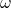. The rotating disk provides energy to the system by the work done by the driving force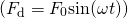. The experimental apparatus is shown in (Figure).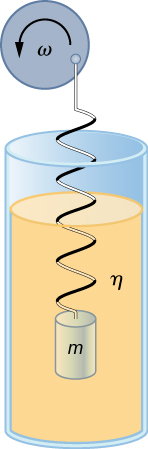Figure 15.30 Forced, damped harmonic motion produced by driving a spring and mass with a disk driven by a variable-speed motor.

Using Newton’s second law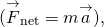we can analyze the motion of the mass. The resulting equation is similar to the force equation for the damped harmonic oscillator, with the addition of the driving force: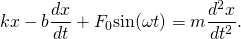When an oscillator is forced with a periodic driving force, the motion may seem chaotic. The motions of the oscillator is known as transients. After the transients die out, the oscillator reaches a steady state, where the motion is periodic. After some time, the steady state solution to this differential equation is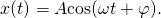Once again, it is left as an exercise to prove that this equation is a solution. Taking the first and second time derivative of x(t) and substituting them into the force equation shows that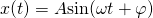is a solution as long as the amplitude is equal to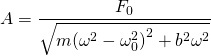where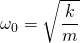is the natural angular frequency of the system of the mass and spring. Recall that the angular frequency, and therefore the frequency, of the motor can be adjusted. Looking at the denominator of the equation for the amplitude, when the driving frequency is much smaller, or much larger, than the natural frequency, the square of the difference of the two angular frequencies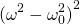is positive and large, making the denominator large, and the result is a small amplitude for the oscillations of the mass. As the frequency of the driving force approaches the natural frequency of the system, the denominator becomes small and the amplitude of the oscillations becomes large. The maximum amplitude results when the frequency of the driving force equals the natural frequency of the system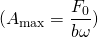.

(Figure) shows a graph of the amplitude of a damped harmonic oscillator as a function of the frequency of the periodic force driving it. Each of the three curves on the graph represents a different amount of damping. All three curves peak at the point where the frequency of the driving force equals the natural frequency of the harmonic oscillator. The highest peak, or greatest response, is for the least amount of damping, because less energy is removed by the damping force. Note that since the amplitude grows as the damping decreases, taking this to the limit where there is no damping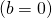, the amplitude becomes infinite.

Note that a small-amplitude driving force can produce a large-amplitude response. This phenomenon is known as resonance. A common example of resonance is a parent pushing a small child on a swing. When the child wants to go higher, the parent does not move back and then, getting a running start, slam into the child, applying a great force in a short interval. Instead, the parent applies small pushes to the child at just the right frequency, and the amplitude of the child’s swings increases.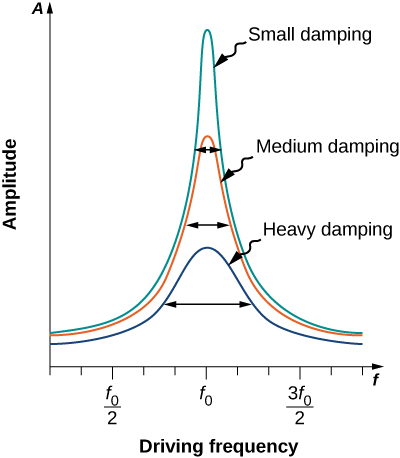Figure 15.31 Amplitude of a harmonic oscillator as a function of the frequency of the driving force. The curves represent the same oscillator with the same natural frequency but with different amounts of damping. Resonance occurs when the driving frequency equals the natural frequency, and the greatest response is for the least amount of damping. The narrowest response is also for the least damping.

It is interesting to note that the widths of the resonance curves shown in (Figure) depend on damping: the less the damping, the narrower the resonance. The consequence is that if you want a driven oscillator to resonate at a very specific frequency, you need as little damping as possible. For instance, a radio has a circuit that is used to choose a particular radio station. In this case, the forced damped oscillator consists of a resistor, capacitor, and inductor, which will be discussed later in this course. The circuit is “tuned” to pick a particular radio station. Here it is desirable to have the resonance curve be very narrow, to pick out the exact frequency of the radio station chosen. The narrowness of the graph, and the ability to pick out a certain frequency, is known as the quality of the system. The quality is defined as the spread of the angular frequency, or equivalently, the spread in the frequency, at half the maximum amplitude, divided by the natural frequency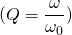as shown in (Figure). For a small damping, the quality is approximately equal to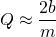.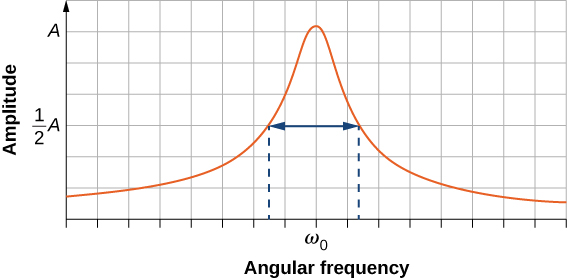Figure 15.32 The quality of a system is defined as the spread in the frequencies at half the amplitude divided by the natural frequency.

These features of driven harmonic oscillators apply to a huge variety of systems. For instance, magnetic resonance imaging (MRI) is a widely used medical diagnostic tool in which atomic nuclei (mostly hydrogen nuclei or protons) are made to resonate by incoming radio waves (on the order of 100 MHz). In all of these cases, the efficiency of energy transfer from the driving force into the oscillator is best at resonance. (Figure) shows a photograph of a famous example (the Tacoma Narrows bridge) of the destructive effects of a driven harmonic oscillation. The Millennium bridge in London was closed for a short period of time for the same reason while inspections were carried out. Observations lead to modifications being made to the bridge prior to the reopening.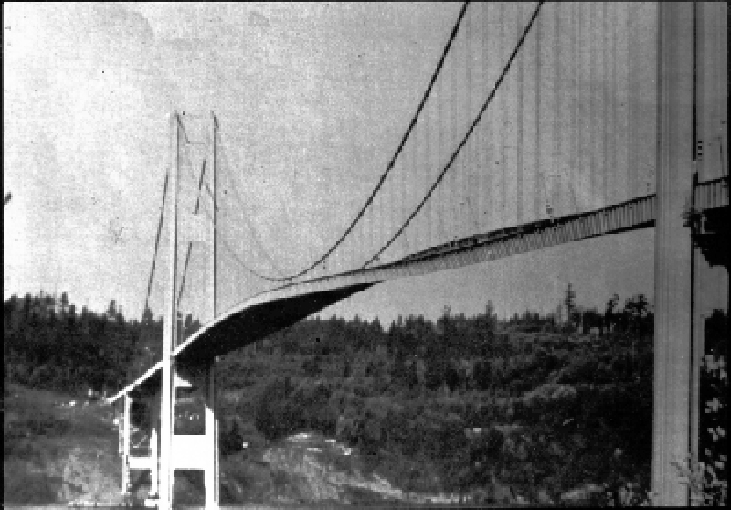Figure 15.33 In 1940, the Tacoma Narrows bridge in the state of Washington collapsed. Moderately high, variable cross-winds (much slower than hurricane force winds) drove the bridge into oscillations at its resonant frequency. Damping decreased when support cables broke loose and started to slip over the towers, allowing increasingly greater amplitudes until the structure failed.

A famous magic trick involves a performer singing a note toward a crystal glass until the glass shatters. Explain why the trick works in terms of resonance and natural frequency.

The performer must be singing a note that corresponds to the natural frequency of the glass. As the sound wave is directed at the glass, the glass responds by resonating at the same frequency as the sound wave. With enough energy introduced into the system, the glass begins to vibrate and eventually shatters.

Summary

• A system’s natural frequency is the frequency at which the system oscillates if not affected by driving or damping forces.
• A periodic force driving a harmonic oscillator at its natural frequency produces resonance. The system is said to resonate.
• The less damping a system has, the higher the amplitude of the forced oscillations near resonance. The more damping a system has, the broader response it has to varying driving frequencies.

Key Equations

 Relationship between frequency and period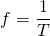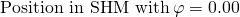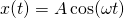General position in SHM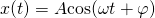General velocity in SHM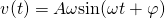General acceleration in SHM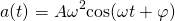Maximum displacement (amplitude) of SHM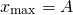Maximum velocity of SHM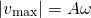Maximum acceleration of SHM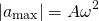Angular frequency of a mass-spring system in SHM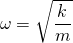Period of a mass-spring system in SHM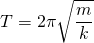Frequency of a mass-spring system in SHM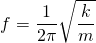Energy in a mass-spring system in SHM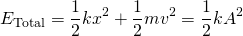The velocity of the mass in a spring-mass system in SHM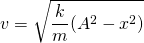The x-component of the radius of a rotating disk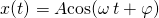The x-component of the velocity of the edge of a rotating disk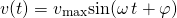The x-component of the acceleration of the edge of a rotating disk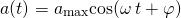Force equation for a simple pendulum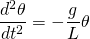Angular frequency for a simple pendulum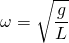Period of a simple pendulum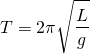Angular frequency of a physical pendulum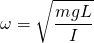Period of a physical pendulum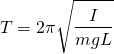Period of a torsional pendulum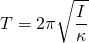Newton’s second law for harmonic motion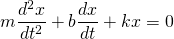Solution for underdamped harmonic motion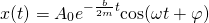Natural angular frequency of a mass-spring systemAngular frequency of underdamped harmonic motion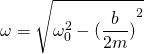Newton’s second law for forced, damped oscillation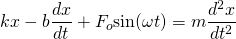Solution to Newton’s second law for forced, damped oscillationsAmplitude of system undergoing forced, damped oscillations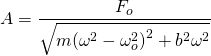Conceptual Questions

Why are soldiers in general ordered to “route step” (walk out of step) across a bridge?

Do you think there is any harmonic motion in the physical world that is not damped harmonic motion? Try to make a list of five examples of undamped harmonic motion and damped harmonic motion. Which list was easier to make?

All harmonic motion is damped harmonic motion, but the damping may be negligible. This is due to friction and drag forces. It is easy to come up with five examples of damped motion: (1) A mass oscillating on a hanging on a spring (it eventually comes to rest). (2) Shock absorbers in a car (thankfully they also come to rest). (3) A pendulum is a grandfather clock (weights are added to add energy to the oscillations). (4) A child on a swing (eventually comes to rest unless energy is added by pushing the child). (5) A marble rolling in a bowl (eventually comes to rest). As for the undamped motion, even a mass on a spring in a vacuum will eventually come to rest due to internal forces in the spring. Damping may be negligible, but cannot be eliminated.

Some engineers use sound to diagnose performance problems with car engines. Occasionally, a part of the engine is designed that resonates at the frequency of the engine. The unwanted oscillations can cause noise that irritates the driver or could lead to the part failing prematurely. In one case, a part was located that had a length L made of a material with a mass M. What can be done to correct this problem?

Problems

How much energy must the shock absorbers of a 1200-kg car dissipate in order to damp a bounce that initially has a velocity of 0.800 m/s at the equilibrium position? Assume the car returns to its original vertical position.

If a car has a suspension system with a force constant of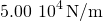, how much energy must the car’s shocks remove to dampen an oscillation starting with a maximum displacement of 0.0750 m?

141 J

(a) How much will a spring that has a force constant of 40.0 N/m be stretched by an object with a mass of 0.500 kg when hung motionless from the spring? (b) Calculate the decrease in gravitational potential energy of the 0.500-kg object when it descends this distance. (c) Part of this gravitational energy goes into the spring. Calculate the energy stored in the spring by this stretch, and compare it with the gravitational potential energy. Explain where the rest of the energy might go.

Suppose you have a 0.750-kg object on a horizontal surface connected to a spring that has a force constant of 150 N/m. There is simple friction between the object and surface with a static coefficient of friction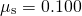. (a) How far can the spring be stretched without moving the mass? (b) If the object is set into oscillation with an amplitude twice the distance found in part (a), and the kinetic coefficient of friction is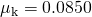, what total distance does it travel before stopping? Assume it starts at the maximum amplitude.

a.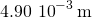; b.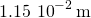Suppose you attach an object with mass m to a vertical spring originally at rest, and let it bounce up and down. You release the object from rest at the spring’s original rest length, the length of the spring in equilibrium, without the mass attached. The amplitude of the motion is the distance between the equilibrium position of the spring without the mass attached and the equilibrium position of the spring with the mass attached. (a) Show that the spring exerts an upward force of 2.00mg on the object at its lowest point. (b) If the spring has a force constant of 10.0 N/m, is hung horizontally, and the position of the free end of the spring is marked as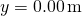, where is the new equilibrium position if a 0.25-kg-mass object is hung from the spring? (c) If the spring has a force constant of 10.0 M/m and a 0.25-kg-mass object is set in motion as described, find the amplitude of the oscillations. (d) Find the maximum velocity.

A diver on a diving board is undergoing SHM. Her mass is 55.0 kg and the period of her motion is 0.800 s. The next diver is a male whose period of simple harmonic oscillation is 1.05 s. What is his mass if the mass of the board is negligible?

Suppose a diving board with no one on it bounces up and down in a SHM with a frequency of 4.00 Hz. The board has an effective mass of 10.0 kg. What is the frequency of the SHM of a 75.0-kg diver on the board?

The device pictured in the following figure entertains infants while keeping them from wandering. The child bounces in a harness suspended from a door frame by a spring. (a) If the spring stretches 0.250 m while supporting an 8.0-kg child, what is its force constant? (b) What is the time for one complete bounce of this child? (c) What is the child’s maximum velocity if the amplitude of her bounce is 0.200 m?

A mass is placed on a frictionless, horizontal table. A spring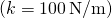, which can be stretched or compressed, is placed on the table. A 5.00-kg mass is attached to one end of the spring, the other end is anchored to the wall. The equilibrium position is marked at zero. A student moves the mass out to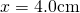and releases it from rest. The mass oscillates in SHM. (a) Determine the equations of motion. (b) Find the position, velocity, and acceleration of the mass at time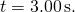Find the ratio of the new/old periods of a pendulum if the pendulum were transported from Earth to the Moon, where the acceleration due to gravity is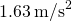.

ratio of 2.45

At what rate will a pendulum clock run on the Moon, where the acceleration due to gravity is, if it keeps time accurately on Earth? That is, find the time (in hours) it takes the clock’s hour hand to make one revolution on the Moon.

If a pendulum-driven clock gains 5.00 s/day, what fractional change in pendulum length must be made for it to keep perfect time?

The length must increase by 0.0116%.

A 2.00-kg object hangs, at rest, on a 1.00-m-long string attached to the ceiling. A 100-g mass is fired with a speed of 20 m/s at the 2.00-kg mass, and the 100.00-g mass collides perfectly elastically with the 2.00-kg mass. Write an equation for the motion of the hanging mass after the collision. Assume air resistance is negligible.

A 2.00-kg object hangs, at rest, on a 1.00-m-long string attached to the ceiling. A 100-g object is fired with a speed of 20 m/s at the 2.00-kg object, and the two objects collide and stick together in a totally inelastic collision. Write an equation for the motion of the system after the collision. Assume air resistance is negligible.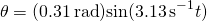Assume that a pendulum used to drive a grandfather clock has a length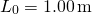and a mass M at temperature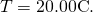It can be modeled as a physical pendulum as a rod oscillating around one end. By what percentage will the period change if the temperature increases by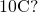Assume the length of the rod changes linearly with temperature, where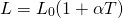and the rod is made of brass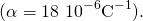A 2.00-kg block lies at rest on a frictionless table. A spring, with a spring constant of 100 N/m is attached to the wall and to the block. A second block of 0.50 kg is placed on top of the first block. The 2.00-kg block is gently pulled to a position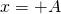and released from rest. There is a coefficient of friction of 0.45 between the two blocks. (a) What is the period of the oscillations? (b) What is the largest amplitude of motion that will allow the blocks to oscillate without the 0.50-kg block sliding off?

a. 0.99 s; b. 0.11 m

Challenge Problems

A suspension bridge oscillates with an effective force constant of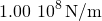. (a) How much energy is needed to make it oscillate with an amplitude of 0.100 m? (b) If soldiers march across the bridge with a cadence equal to the bridge’s natural frequency and impart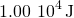of energy each second, how long does it take for the bridge’s oscillations to go from 0.100 m to 0.500 m amplitude.

Near the top of the Citigroup Center building in New York City, there is an object with mass of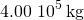on springs that have adjustable force constants. Its function is to dampen wind-driven oscillations of the building by oscillating at the same frequency as the building is being driven—the driving force is transferred to the object, which oscillates instead of the entire building. (a) What effective force constant should the springs have to make the object oscillate with a period of 2.00 s? (b) What energy is stored in the springs for a 2.00-m displacement from equilibrium?

a.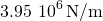; b.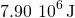Parcels of air (small volumes of air) in a stable atmosphere (where the temperature increases with height) can oscillate up and down, due to the restoring force provided by the buoyancy of the air parcel. The frequency of the oscillations are a measure of the stability of the atmosphere. Assuming that the acceleration of an air parcel can be modeled as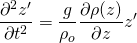, prove that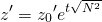is a solution, where N is known as the Brunt-Väisälä frequency. Note that in a stable atmosphere, the density decreases with height and parcel oscillates up and down.

Consider the van der Waals potential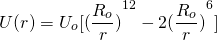, used to model the potential energy function of two molecules, where the minimum potential is at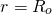. Find the force as a function of r. Consider a small displacement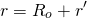and use the binomial theorem: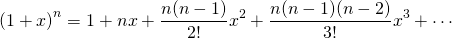,

to show that the force does approximate a Hooke’s law force.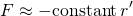Suppose the length of a clock’s pendulum is changed by 1.000%, exactly at noon one day. What time will the clock read 24.00 hours later, assuming it the pendulum has kept perfect time before the change? Note that there are two answers, and perform the calculation to four-digit precision.

(a) The springs of a pickup truck act like a single spring with a force constant of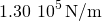. By how much will the truck be depressed by its maximum load of 1000 kg? (b) If the pickup truck has four identical springs, what is the force constant of each?

a. 7.54 cm; b.